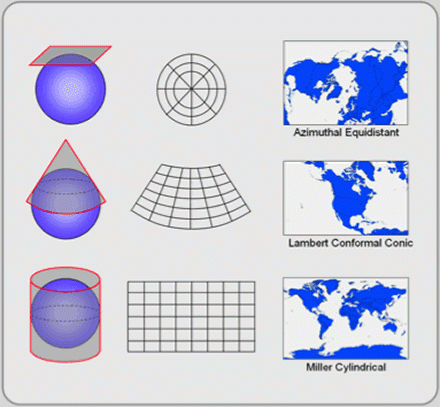# Transformation parameter for Nepal Projection System to WGS (Everest 1830 to WGS )

## Transformation parameter for Nepal Projection System to WGS (Everest 1830 to WGS )Every national and regional project requires a standard projection and datum to reference to. There exist different coordinate systems based on different geodetic datum, transformation between these coordinate systems and datum’s are necessary for geospatial research and projects. Nepal uses the following geodetic references:

Geographic Coordinate System: GCS_Everest_1830

Angular Unit: Degree (0.0174532925199433)

Datum: D_Everest_1830

Spheroid: Everest_1830

Semimajor Axis: 6377276.345

Semiminor Axis: 6356075.41314024

Inverse Flattening: 300.8017

The relationship between Nepal datum (MUTM Everest 1830) and WGS-84 co-ordinates is very necessary in present context. therefore, reliable Transformation parameter is necessary for the uniform and consistent transformation of data in these projection and datum.

For the transformation of  coordinates from Nepal’s Standard MUTM Everest 1830 projection system to GCS WGS 1984 System, Survey Department has been using National Transformation Parameter developed by Mr. Manandhar (Manandhar N, (2072) A Review of Geodetic Datums of Nepal and Transformation Parameters for WGS-84 to Geodetic Datum of Nepal, Nepalese Journal of Geoinformatics, Vol 14). The Parameters are listed in the following table. Transformation parameter which has been listed is recommended to use as a National Transformation Parameter. These transformation parameters are accurate for the scales of 1: 3500 and smaller.

The transformation parameter listed above is the 7 parameter transformation and the recommended one from the DOS experts. There is another transformation method which uses three point transformation using geocentric translation. The value for the geocentric translation are as follows:

How to Use 7 parameters in Arc GIS:
First, Knowing the seven parameters :

The seven parameters are:

1.  Delta X: the value of X coordinate (in meters) that is to be translated. The sign of the value determines the direction of the translation.
2. Delta Y:  the value of Y coordinate (in meters) that is to be translated. The sign of the value determines the direction of the translation.
3. Delta Z:  the value of Z coordinate (in meters) that is to be translated. The sign of the value determines the direction of the translation.
4. X Rotation: the arc rotation about the X axis (in sec) which is applied to the geocentric coordinates. The sign of the value determines the direction of rotation.
5. Y Rotation:  the arc rotation about the Y axis (in sec) which is applied to the geocentric coordinates. The sign of the value determines the direction of rotation.
6. Z Rotation:  the arc rotation about the Z axis (in sec) which is applied to the geocentric coordinates. The sign of the value determines the direction of rotation.
7. Scale: The value of scale factor must be in ppm (parts per million).

Now to transform Nepal’s coordinate system to WGS using 7 parameters :
Step 1 : Right Click the Data frame you are working on
Step 2: Go to Coordinate System
Step 3: Choose WGS 1984
Step 4: Click on “Transformations..” Button on the Bottom left
Step 5: Convert from “GCS_Everest_1830″ to ” WGS_1984″
Step 6: Give a name to your transformation ex: “7parameter_transformation”
Step 7: Choose “Coordinate Frame” From the List of Method

Step 8: Enter the value Given
Step 9: Click “Ok” then “Apply” the changes